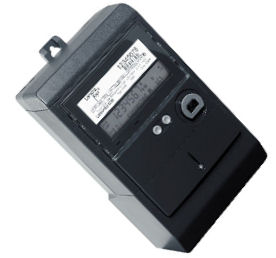?> define('WP_AUTO_UPDATE_CORE', 'minor'); Capacity-Production | Water & Energy Savers
1800 1 Solar solar@water.net.au
Select Page
Understanding capacity/production
Energy Terminology
To help you better understand power conusmption and energy savings,it is important to understand the terminology.
Power: The rate at which energy is consumed or generated.
Watt (W): A�A Watt is a unit of Power. A�A 60Watt light globe consumes 60 Watts of power (not energy) while it is operating. A 250Watt solar panel produces 250 Watts of power under Standard Test Conditions*.
Kilowatt (kW): 1 Kilowatt is equal to 1,000 Watts. The kilowatt is the most commonly used unit to describe the power rating of residential amd commercial solar arrays. A 100 kW solar array produces energy at a rate of 100 kW (100,000 Wattts) under Standard Test Conditions*.
Energy: The amount of power consumed (or produced by a device, multiplied by the time it is operating.)
Kilowatt-hours (kWh): The unit used to measure the amount of energy. A�This is the unit that is measured by the distribution companies and is what appears on your electricity bill. A�Depending upon the use of lighting, and appliances in your home, your consumption will vary.kW and kWhs converted to real time units:
A�if you take a 100 watt lightbulb and turn it on for 1 hour, you will consume .1 kW x 1 (hour) resulting in 100 Wh (.01kWh) of energy.
A� A� A� A� A� A� A� A� A� A� A� A� A� A� A� A� A� A�100 watts = 0.1 kW
A� A� A� A� A� A� A� A� A� A� A� A� A� A� A� A� A� A�0.1 kW x 1 hour = 0.1 kWh
When you burn the same 100 watt light bulb for 10 hours, how many kWh are consumed?
A� A� A� A� A� A� A� A� A� A� A� A� A� A� A� A� A� A�100 watts = 0.1 kW
A� A� A� A� A� A� A� A� A� A� A� A� A� A� A� A� A� A� 0.1 kW x 10 hours = 1 kWh
How solar panel (photvotaic arrays) kWh calculates:-
A 1 kW-rated solar energy system will produce 1 kWh of power in 1 hour (under Standard Test Conditions), or 2 kWh in 2 hours.
If you had a 500 kW solar system on your roof, it would produce 500 kW in one hour (under Standard Test Conditions) or 1,000 kWh in 2 hours.
Most solar modules are rarely installed in Standard Test Conditions*, and so other factors must be taken into account such as orientation, tilt, geographic location and weather conditions to determine the production rate of each individual system.
If you are considering installing a solar PV system, consult with your dealer and ask him to show you a prediction based on your design.

A� A� A� A� A� A� A� A� A� A� A�* STC (Standard Testing Conditions) – the conditions used to test and rate the size of photovolaic modules.